Home
Hostname: page-component-568f69f84b-klmjj Total loading time: 0.244 Render date: 2021-09-21T21:33:04.593Z Has data issue: true Feature Flags: { "shouldUseShareProductTool": true, "shouldUseHypothesis": true, "isUnsiloEnabled": true, "metricsAbstractViews": false, "figures": true, "newCiteModal": false, "newCitedByModal": true, "newEcommerce": true, "newUsageEvents": true }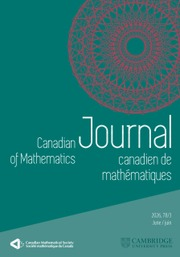Canadian Journal of Mathematics

# BMO Functions and Carleson Measures with Values in Uniformly Convex Spaces

Published online by Cambridge University Press:  20 November 2018

## Abstract

HTML view is not available for this content. However, as you have access to this content, a full PDF is available via the ‘Save PDF’ action button.

This paper studies the relationship between vector-valued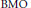$\text{BMO}$ functions and the Carleson measures defined by their gradients. Let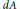$dA$ and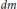$dm$ denote Lebesgue measures on the unit disc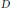$D$ and the unit circle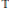$\mathbb{T}$ , respectively. For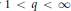$1\,<\,q\,<\,\infty$ and a Banach space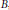$B$ , we prove that there exists a positive constant$c$ such that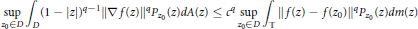$$\underset{{{z}_{0}}\in D}{\mathop{\sup }}\,{{\int }_{D}}{{\left( 1-\left| z \right| \right)}^{q-1}}{{\left\| \nabla f\left( z \right) \right\|}^{q}}{{P}_{{{Z}_{0}}}}\left( z \right)dA\left( z \right)\le {{c}^{q}}\underset{{{z}_{0}}\in D}{\mathop{\sup }}\,{{\int }_{\mathbb{T}}}{{\left\| f\left( z \right)-f\left( {{z}_{0}} \right) \right\|}^{q}}{{P}_{{{z}_{0}}}}\left( z \right)dm\left( z \right)$$

holds for all trigonometric polynomials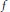$f$ with coefficients in$B$ if and only if$B$ admits an equivalent norm which is$q$ -uniformly convex, where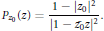$${{P}_{{{z}_{0}}}}\left( z \right)=\frac{1-|{{z}_{0}}{{|}^{2}}}{|1-{{{\bar{z}}}_{0}}z{{|}^{2}}}.$$

The validity of the converse inequality is equivalent to the existence of an equivalent$q$ -uniformly smooth norm.

## Keywords

Type
Research Article
Information
Canadian Journal of Mathematics , 01 August 2010 , pp. 827 - 844

## References

 Blasco, O., Hardy spaces of vector-valued functions: duality. Trans. Amer. Math. Soc. 308(1988), no. 2, 495–507. doi:10.2307/2001088Google Scholar
 Blasco, O., Remarks on vector-valued B MOA and vector-valued multipliers. Positivity 4(2000), no. 4, 339–356. doi:10.1023/A:1009890316575Google Scholar
 Bourgain, J., Vector-valued singular integrals and the H1-B MO duality. In: Probability theory and harmonic analysis, Monogr. Textbooks Pure Appl. Math., 98, Dekker, New York, 1986, pp. 1–19.Google Scholar
 Coifman, R. R., Meyer, Y., and Stein, E. M., Some new function spaces and their applications to harmonic analysis. J. Funct. Anal. 62(1985), no. 2, 304–335. doi:10.1016/0022-1236(85)90007-2Google Scholar
 Garćıa-Cuerva, J. and Rubio de Francia, J. L., Weighted norm inequalities and related topics. North-Holland Mathematics Studies, 116, North-Holland Publishing Co., Amsterdam, 1985.Google Scholar
 Garnett, J. B., Bounded analytic functions. Pure and Applied Mathematics, 96, Academic Press Inc., New York–London, 1981.Google Scholar
 Kwapień, S., Isomorphic characterizations of inner product spaces by orthogonal series with vector valued coefficients. Studia Math. 44(1972), 583–595.Google Scholar
 Lindenstrauss, J. and Tzafriri, L., Classical Banach spaces. II. Function spaces. Results in Mathematics and Related Areas, 97, Springer-Verlag, Berlin–New York, 1979.CrossRefGoogle Scholar
 Martınez, T., Torrea, J. L., and Xu, Q., Vector-valued Littlewood–Paley–Stein theory for semigroups. Adv. Math. 203(2006), no. 2, 430–475. doi:10.1016/j.aim.2005.04.010Google Scholar
 Pisier, G., Martingales with values in uniformly convex spaces. Israel J. Math. 20(1975), no. 3–4, 326–350. doi:10.1007/BF02760337Google Scholar
 Pisier, G., Probabilistic methods in the geometry of Banach spaces. In: Probability and analysis, Lecture Notes in Math., 1206, Springer, Berlin, 1986, pp. 167–241.Google Scholar
 Pisier, G., Factorization of linear operators and geometry of Banach spaces. CB MS Regional Conference Series in Mathematics, 60, American Mathematical Society, Providence, RI, 1986.CrossRefGoogle Scholar
 Stein, E. M., Singular integrals and differentiability properties of functions. Princeton Mathematical Series, 30, Princeton University Press, Princeton, NJ, 1970.Google Scholar
 Stein, E. M., Harmonic analysis: real-variable methods, orthogonality, and oscillatory integrals. Princeton Mathematical Series, 43, Princeton University Press, Princeton, NJ, 1993.Google Scholar
 Xu, Q., Littlewood–Paley theory for functions with values in uniformly convex spaces. J. Reine Angew. Math. 504(1998), 195–226. doi:10.1515/crll.1998.107Google ScholarYou have Access
4
Cited by

# Send article to Kindle

Note you can select to send to either the @free.kindle.com or @kindle.com variations. ‘@free.kindle.com’ emails are free but can only be sent to your device when it is connected to wi-fi. ‘@kindle.com’ emails can be delivered even when you are not connected to wi-fi, but note that service fees apply.

Find out more about the Kindle Personal Document Service.

BMO Functions and Carleson Measures with Values in Uniformly Convex Spaces
Available formats
×

# Send article to Dropbox

To send this article to your Dropbox account, please select one or more formats and confirm that you agree to abide by our usage policies. If this is the first time you use this feature, you will be asked to authorise Cambridge Core to connect with your <service> account. Find out more about sending content to Dropbox.

BMO Functions and Carleson Measures with Values in Uniformly Convex Spaces
Available formats
×

# Send article to Google Drive

To send this article to your Google Drive account, please select one or more formats and confirm that you agree to abide by our usage policies. If this is the first time you use this feature, you will be asked to authorise Cambridge Core to connect with your <service> account. Find out more about sending content to Google Drive.

BMO Functions and Carleson Measures with Values in Uniformly Convex Spaces
Available formats
×
×